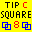Square Rotation Program (SQROT)

SQROT 5.44  (August 2019)

How rotations are generated by theSquare Rotation Program.

For each tip, the program proceeds as follows:
1. If the number of 'ready' couples is not a multiple of 4, the program determines who will sit out by selecting couples who have sat out the fewest number of times, using a ratio of number of tips danced vs. number of tips sat out. If several couples have the same ratio, couples to sit out will be selected at random from those couples.
2. A random rotation is generated.
3. The following procedure is repeated until no more changes are possible or until the user clicks the 'abort process' button:
1. For each pair of couples, a value is calculated indicating how often those two couples have danced together. This value is then subtracted from the optimal number of times that the couples should have danced together. The resulting value is then squared.
2. All values are added together to produce a 'chi-square-like' statistic.
3. Two couples (in different squares) are swapped within the rotation and the chi-square statistic is recalculated. If the new statistic is less than the old statistic, the new rotation is saved, otherwise, the previous rotation is restored.
4. The process continues until the program has tried swapping every couple with every other couple and no decrease has occurred within the statistic.
4. Square numbers are then assigned and 'optimized' in a similar manner (also using a 'chi-square' statistic) so that dancers move around between different squares on the floor.

https://www.ceder.net/sqrot/sqrot_tech.php
09-May-2021 17:53:02